## Introduction to Fractions

Practice Unlimited Questions

#### 1. What is a fraction?

A fraction is a part of a whole.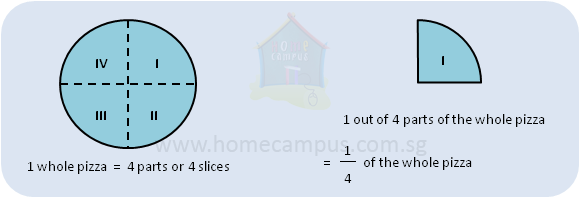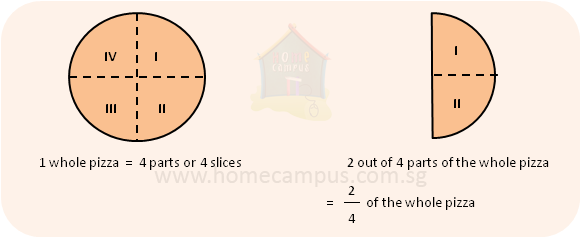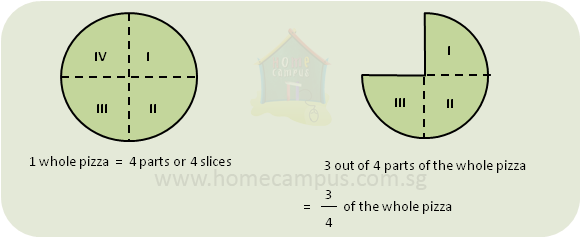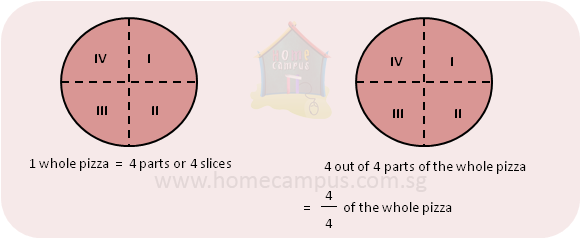#### 2. What are numerator and denominator?

A fraction has got two numbers.
The top number is called the numerator.
The bottom number is called the denominator.

#### 3. Annie, Betty and Charlie shared a pizza that was cut into 4 slices. Annie got 1 slice, Betty got 2 slices and Charlie got 1 slice. What fraction of the pizza did each of them receive?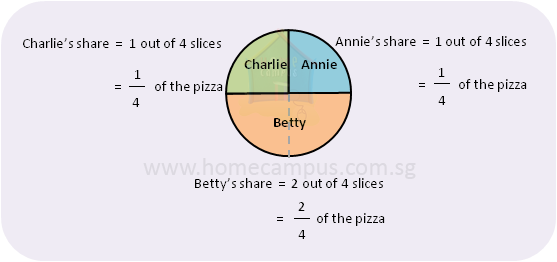1 4
is read as "1 upon 4" or "1 over 4" or "1 out of 4".

Annie:
So, her share of the pizza was
 1 4
.
The numerator, which is 1, tells us how many slices Annie got.
The denominator, which is 4, tells us how many equal slices the pizza was cut into.
Betty:
Betty received 2 slices of the pizza.
Betty's share of the pizza was
 2 4
.
 2 4
can be simplified to
 1 2
.
So, Betty's share of the pizza was
 2 4
or
 1 2
.
Charlie:
Charlie received 1 out of the 4 slices that the pizza was cut into.
Charlie's share of the pizza was
 1 4
.